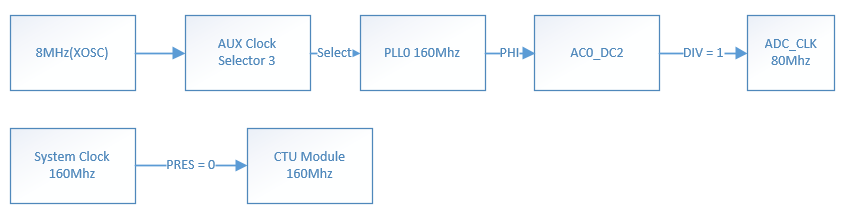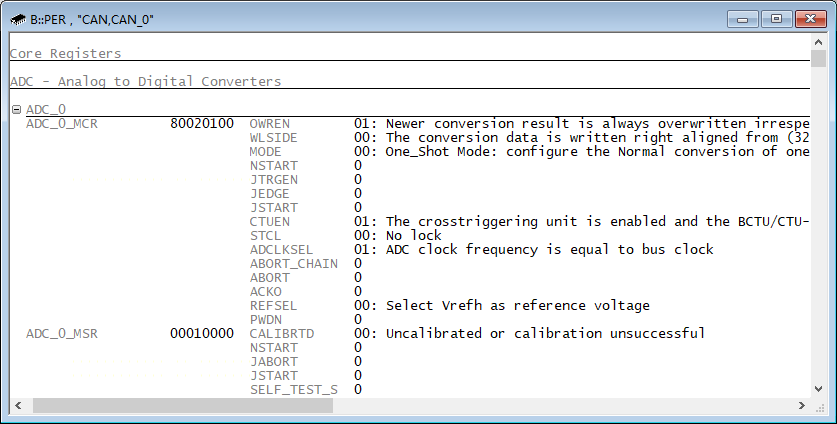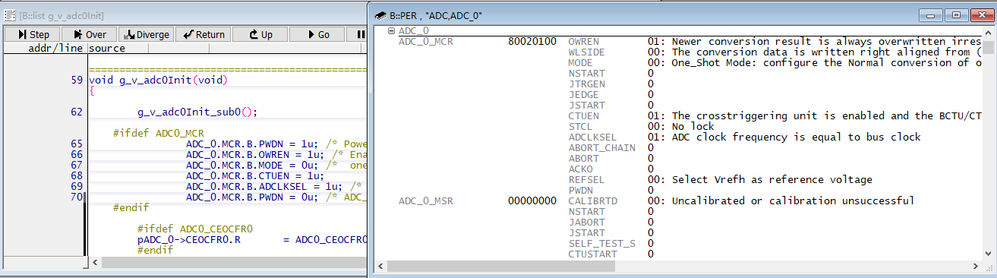## How to design the reserved time of MPC5741P adjacent CTU Trigger to avoid the declaration of ADC_OE?

cancel
Showing results for
Search instead for
Did you mean:

## How to design the reserved time of MPC5741P adjacent CTU Trigger to avoid the declaration of ADC_OE?

262 ViewsContributor I

Dear,

We have a question at present: why should we leave at least 2.25us after one CTU Trigger triggers a set of ADC command list, and the other Trigger triggers the next sampling command to be normal, otherwise an ADC_OE error will be reported?According to the theory described on UM, if each CTU only triggers a set of AD sampling time of 275ns+750ns, it only needs about 1us.

Labels (1)
• ### General

Tags (3)
7 Replies
130 ViewsNXP TechSupport

Hi,

The ADC_OE is set when new command for the ADC occurs while previous triggered conversion is not finished. So you should check the command list and triggering scheme to be sure the new command is not issued before previous conversion finish.

The ADC conversion time is discussed in the chapter 36.5.11 Conversion time of the Reference Manual.

BR, Petr

130 ViewsContributor I

Hi,

Thank you very much for your reply. In my question above, 275ns+750ns is calculated according to chapter 36.5.11 Conversion time in UM, so we think that the failure of ADC_OE can be avoided only if the distance is more than 1.025us, but the distance measured should be at least 2.25us, so we don't know why the theoretical value and the actual test value differ so much?

130 ViewsNXP TechSupport

Hi,

hard to say without knowledge of your setting. So can you share the clocking option and CTU/ADC initialization?

BR, Petr

130 ViewsContributor I

Hi

Take the following example to describe the problems we encountered:ADC Initialization function Key register configuration: (The sampling channel is normally enabled)

pADC_0->CALBISTREG.R = 0xC03706F0U;

pADC_0->MCR.R = 0x00000001U;

pADC_0->MCR.R = 0x80020100U;

pADC_0->CTR0.R = 0x00000016U;

pADC_0->CTR1.R = 0x00000016U;

CTU Initialization function Key register configuration:

pCTU_0->TGSISR.R = 0x00000001u;

pCTU_0->TGSCR.R = 0x0100u;

pCTU_0->TCR.R = 0xC8u;

pCTU_0->TCR.R = 0x2A8u;

pCTU_0->TGSCCR.R = 0x7fffu;

pCTU_0->CLCR1.R = 0x00000300u;

pCTU_0->THCR1.R = 0x00006161u;

pCTU_0->CLR.B.R = 0x20C8u;

pCTU_0->CLR.B.R = 0x206Eu;

pCTU_0->CLR.B.R = 0x6000u;

pCTU_0->CLR.B.R = 0x20C8u;

pCTU_0->CLR.B.R = 0x6000u;

pCTU_0->FTH.R =0x00000005u;

pCTU_0->COTR.R = 0x0000u;

pCTU_0->CR.R = 0x00000011u;

The above is a simple initialization configuration for the registers of the two modules

Trigger0 is set to 1.25us, and trigger1 is set to 4.25us, with a difference of 3us.

Refer to chapter 36.5.11 Conversion time in UM for the calculation formula of sample Conversion time

• CALBISTREG[OPMODE] = 000b; CTRx[INPSAMP] = 22d; CTU triggered:

• Single/First Conversion: 2 + 22 + ((13 + 1) × 4) + 2 = 2 + 22 + 56 + 2 = 82cycles of AD_clk

• Subsequent/continuous conversion: 1 + 22 + ((13 + 1) × 4) + 2 =1 + 22 + 56 + 2 = 81 cycles of AD_clk

The conversion time required to sample two ADC command lists is 82+81 ADC CLKS = 2.0375us

When the trigger is designed above, the time interval is 3us. If calculated according to the time provided in UM, ADC_OE error will not be reported, but actually ADC_OE error will be reported. The ADC_OE error will not be reported  When trigger1 time is greater than 5.7us.So the question is right here, why did the design report error according to the instructions given by UM

130 ViewsNXP TechSupport

Hi,

the calculation look correct and the timing could be right. Anyway it seems some clock could be twice less, so I can recommend to double check if the CTU and ADC clocks are as you stated. Is the ADCLKSEL still set?

If you enabled CTU trigger output you can check on the pin the trigger generation, to see the timing.

Finally, you selected dual conversion, however no setting for ADC1 is mentioned. Is this the same as for ADC0?

BR, Petr

130 ViewsContributor I

Hi,

Petr,According to your prompt, I have paid attention to the configuration of ADC clock again.

In my code, the operation on ADCLKSEL was implemented directly by operating the MCR register,

pADC_0->MCR.R = 0x00000001U;

pADC_0->MCR.R = 0x80020100U;

and then I also debugged with the debugger, which showed that the value of the ADCLKSEL bit in the MCR register was 1.However, through a series of experiments, I found that my ADC clock was configured as half bus clock(40MHz).

Then, I changed the register configuration to:

ADC_0.MCR.B.PWDN = 1u;

ADC_0.MCR.B.OWREN = 1u;

ADC_0.MCR.B.MODE = 0u;

ADC_0.MCR.B.CTUEN = 1u;

ADC_0.MCR.B.ADCLKSEL = 1u;

ADC_0.MCR.B.PWDN = 0u;

The debugger debugged the results:According to this code design, the tested ADC clock is 80Mhz, and the time to sample a set of AD data is consistent with the theoretical value of 1us.

Therefore, I would like to ask if there is any way for 5741 chip to determine whether ADCLKSEL is written and effective.Because from my experiment, the debugger didn't look right.

130 ViewsNXP TechSupport

Hi,

MCR[ADCLKSEL] can only be written in power-down, when MCR[PWDN] = 1. So the second code ensures that, I think.

You can try to enable DUALPORT in Lauterbach and see a difference.

BR, Petr